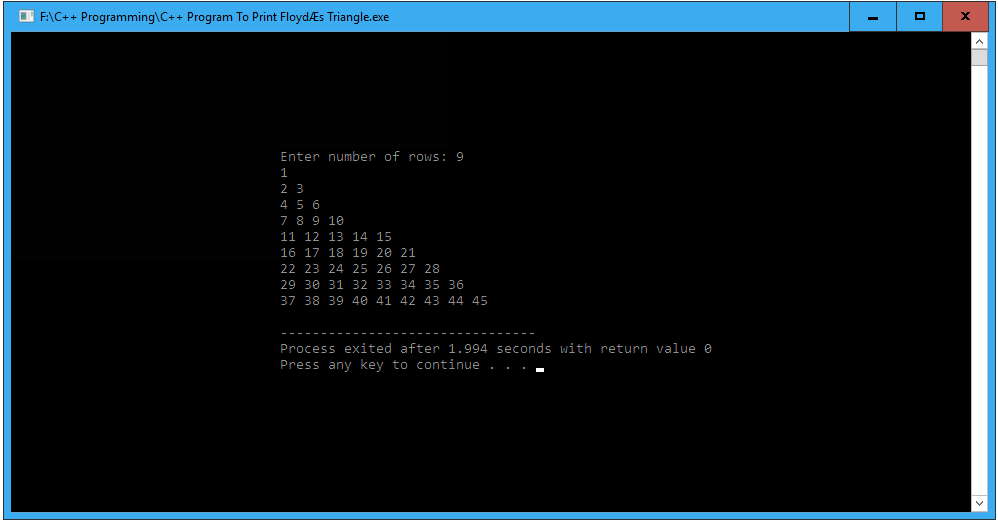# C++ program to print Floyd’s triangle

In this article write a C++ Program to print Floyd’s triangle. This Program first takes the numbers of rows and uses nested for loops to print Floyd’s triangle.

## C++ Program to print Floyd’s triangle

```//C++ program to print Floyd's triangle

#include <iostream>
using namespace std;

int main()
{
int rows, num = 1;

cout << "Enter number of rows: ";
cin >> rows;

// outer loop is responsible for row
for(int i = 1; i <= rows; i++)
{

//inner loop is responsible for columns
for(int j = 1; j <= i; j++)
{

// printing number
cout << num << " ";
num++;
}

// give line breaks after ending every row
cout << endl;
}

return 0;
}
```

### When the above C++ program is compile and run, this will produce the following result: